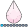NKormanik
Barite | Level 11

## pointlabel -- needs to be cut short, with int()

``````symbol1 pointlabel = ("#N" h=.5 font=simplex nodropcollisions) value=none;

``````

N is a number of cases.  I'd like to see that number as label in a scatterplot, instead of a dot.

``````proc gplot data=have;
plot X * Y = 1;  /* Surmised that "= 1" means symbol1 */
run;
quit;
``````

Unfortunately N shows in the plot having many decimal places, all 0.

I figured using int() would do the trick, to just show N alone, no superfluous unwanted decimal places.

I tried several ways, but none seem to work.  One approach that does not work is:

``"#int(N)"``

Another possibility would be to create an ad-hoc variable, N2, that is int(N), and then to use N2 instead of N in the pointlabel.  But I'm not sure how to code such.

Any help toward a solution greatly appreciated.

Nicholas Kormanik

1 ACCEPTED SOLUTION

Accepted SolutionsDanH_sas
SAS Super FREQ

## Re: pointlabel -- needs to be cut short, with int()

Use the FORMAT statement:

``````proc sgplot data=Have;format N f2.0;
scatter x=x y=y / markerchar=N markercharattrs=(size=14);
run;``````
6 REPLIES 6

## Re: pointlabel -- needs to be cut short, with int()

Would you be willing to use PROC SGPLOT instead?  If so, you can use the MARKERCHAR= option to specify a variable name. Instead of markers, the value of that variable is plotted. You can controls the size and color of the text by using the MARKERCHARATTRS= option:

``````data Have;
input x y N;
datalines;
1 2 37
3 1 23
5 2 16
4 1  2
5 4  1
3 3 18
;

proc sgplot data=Have;
scatter x=x y=y / markerchar=N markercharattrs=(size=14);
run;``````NKormanik
Barite | Level 11

## Re: pointlabel -- needs to be cut short, with int()

Beautiful.  Still, however, all those .000000 after each number in plot.

Got error message when I tried:

``markerchar=int(N)``

and

``markerchar=(int(N))``

Perhaps I should format the N column itself?

Thanks much!Jay54
Meteorite | Level 14

## Re: pointlabel -- needs to be cut short, with int()

Yes, apply the format to the column.  If you don't want decimals, use the x.0 format.  If you review the doc, you will see that MARKERCHAR only accepts a variable, not an expression.  If you want to use expressions, use GTL.NKormanik
Barite | Level 11

## Re: pointlabel -- needs to be cut short, with int()

What's the easiest way to apply that format to the column?DanH_sas
SAS Super FREQ

## Re: pointlabel -- needs to be cut short, with int()

Use the FORMAT statement:

``````proc sgplot data=Have;format N f2.0;
scatter x=x y=y / markerchar=N markercharattrs=(size=14);
run;``````NKormanik
Barite | Level 11

## Re: pointlabel -- needs to be cut short, with int()

Excellent.  This approach has the added benefit of not having to affect/rewrite the dataset.

Thanks much to all of you!

Discussion stats
• 6 replies
• 1229 views
• 2 likes
• 4 in conversation